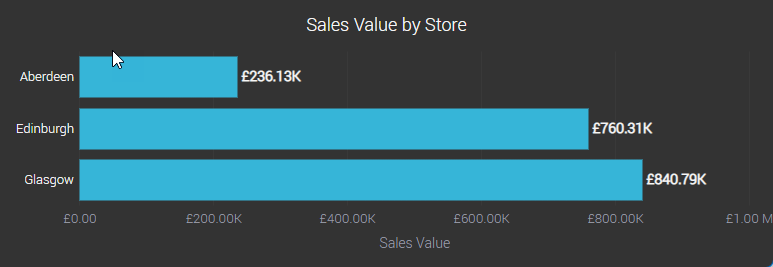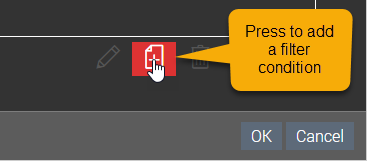### Understanding summary calculations

When we add a metric to a dashboard or visualisation, the value will be calculated by applying some summary operation to each row that matches the dimensions displayed. For instance, in the chart below, the Sales Value is calculated by summing the metric Sales Value in every row that matches a particular store. A filter is applied to the chart so we only see sales for the current year.However, it’s likely we might want to compare sales for each store in the current year with sales in the same stores last year. Summary calculations allow us to achieve this by creating metrics that have an implicit filter applied to them.

For instance, we could create two new metrics Sales TY, which would always only total sales in the current year, and Sales LY which would always only total sales in the previous year.

The chart below has no filters applied, however there are two implicit filters applied in the two metrics Sales TY and Sales LY### Creating summary calculations

To create a summary calculation for Sales TY, follow these steps:2. Select Subjects in the left-hand tab, then find the subject you want to add the summary calculations to and press the edit button.3. Press the Add metric button4. Select the option Create a new metric in the subject from a new calculated column
5. Enter a name the column (we’ll use Sales LY)
6. Select Summary Calculation as the type of calculation
7. Select the column we want to sum (we’ll select Sales Value)
8. Select the summary operation (we’ll use Sum)
9. Define the filter to apply to this summary column. For our Sales LY example, we’ll do the following...
1. Press the Add Filter Condition button to add a filter condition2. Select Date as the column to filter on
3. Select >= as the operator
4. Under the Value heading, select Calendar Value
5. Select Start of this year as of 1 year ago for the calendar value options
6. Press OK7. Repeat steps a and b above to add a second condition.
Select <= as the operator
8. Under the Value heading, select Calendar Value
9. Select End of this year as of 1 year ago for the calendar value options
10. Press OK

### Using summary calculations

Once you have created a summary calculation, it’s available in visualisations and dashboards as any other metric. The implicit filter is automatically applied to any figure where the summary calculation is used.